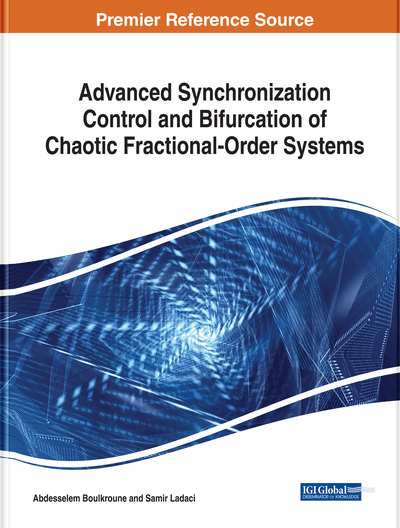# Dynamics and Synchronization of the Fractional-Order Hyperchaotic System

Shaobo He (Hunan University of Arts and Science, China), Huihai Wang (Central South University, China) and Kehui Sun (Central South University, China)
Copyright: © 2018 |Pages: 31
DOI: 10.4018/978-1-5225-5418-9.ch002

## Abstract

The fractional-order Lorenz hyperchaotic system is solved as a discrete map by applying Adomian decomposition method (ADM). Dynamics of this system versus parameters are analyzed by LCEs, bifurcation diagrams, and SE and C0 complexity. Results show that this system has rich dynamical behaviors. Chaos and hyperchaos can be generated by decreasing the fractional derivative order q in this system. It also shows that the system is more complex when q takes smaller values. Moreover, coupled synchronization of fractional-order chaotic system is investigated theoretically. The synchronization performances with synchronization controller parameters and derivative order varying are analyzed. Synchronization and complexity of intermediate variables which generated by ADM are investigated. It shows that intermediate variables between the driving system and response system are synchronized and higher complexity values are found. It lays a technical foundation for secure communication applications of fractional-order chaotic systems.
Chapter Preview
Top

## Introduction

In recent years, dynamical analyses of fractional-order chaotic systems have become a hot topic (Pham et al., 2017; Matouk & Elsadany, 2016; Sun et al., 2010). Fractional-order systems based secure communication and information encryption also aroused researchers’ interests (Chen et al., 2013; Wang et al., 2013; Wang et al., 2014). Currently, three numerical solution algorithms, namely, frequency domain method (FDM) (Charef et al., 1992), Adams-Bashforth-Moulton algorithm (ABM) (Sun et al., 1984) and Adomian decomposition method (ADM) (Adomian, 1990), are mainly used to solve fractional-order chaotic systems. Meanwhile, Wang and Yu (2012) applied multistage homotopy-perturbation method and Arena et al. (2012) employed differential transform method to solve fractional-order chaotic systems. Obviously, the accuracy of different numerical algorithms is different, and the reliability of these algorithms should be investigated. For instance, Tavazoei et al. (2007) reported that FDM is not always reliable in detecting chaotic behaviors in nonlinear systems. Moreover, it is not difficult to find out that by applying different numerical algorithms, dynamical analysis results of fractional-order chaotic systems are different. Take fractional-order Chen system as an example, Tavazoei et al. (2007) showed that the minimum order to generate chaos by ABM is q=0.83 while solved by FDM, the system can generate chaos at q=0.2 and Cafagna and Grassi (2008) showed that chaos can be found even for q=0.08 when the system is analyzed by employing ADM. It is important to compare different solving algorithms and to figure out how to choose a proper algorithm in different application fields by analyzing their characteristics, including speed, computing precision, time and space complexity of different algorithms.

Lyapunov Characteristic Exponents (LCEs) are necessary and more convenient for detecting hyperchaos in fractional-order hyperchaotic system. Li et al. (2010) proposed a definition of LCEs for fractional differential systems based on a frequency-domain approximation, but the limitations of frequency-domain approximations are highlighted by Tavazoei et al. (2007). Time series based LCEs calculation methods like Wolf algorithm which is designed by Wolf et al. (1985), Jacobian method proposed by Ellner et al. (1991) and neural network algorithm presented by Maus and Sprott (2013) have difficulties when choosing the embedding dimension and the delay parameter inherent of the phase-space reconstruction. Recently, LCEs of fractional-order chaotic systems are calculated based on ADM by applying QR decomposition method (Caponetto and Fazzino, 2013). Currently, calculating LCEs of fractional-order chaotic systems versus their parameters and fractional order q has aroused people’s interest.

## Complete Chapter List

Search this Book:
Reset